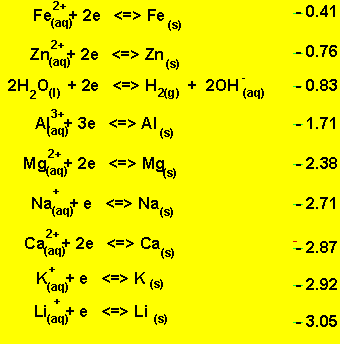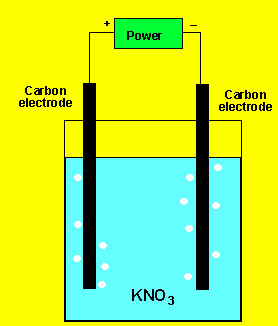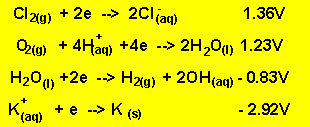Electrolysis in solutions In both electrolytic and electrochemical cells it is the strongest oxidant and the strongest reductant present that will react. Keeping this in mind why is it that molten NaCl is electrolysed rather than a solution of NaCl? Surely it would be a great deal cheaper to feed in brine instead of supplying energy to melt the solid NaCl. Look at the electrochemical series on the right. A solution of NaCl contains the following oxidants Sodium ions and water. Looking at the electrochemical series on the right it is obvious that water is the strongest oxidant present. Water will be reduced at the cathode (-) in preference to the sodium ions. The products at the cathode will be hydrogen gas and hydroxide ions according to the equation below. 2H2O(l) + 2e => H2(g) + 2OH-(aq).Example 1) A solution of potassium chloride was electrolysed as shown on the left. A gas was produced at each electrode but no metal was deposited. Below are the half cell potentials of a select number of half cells. Explain what the gases could be.Competition at the electrodes means that the strongest oxidant and the strongest reductant present will react. The gas at the cathode(-) was collected and tested. Click to see the test. The gas at the anode was collected Click to see the test. Can you identify the gasses? The oxidants present are potassium ions and water. According to the half cell potential water is the strongest oxidant present. So the products formed at the cathode(-) are hydrogen gas and hydroxide ions according to the reaction below. 2H2O(l) + 2e => H2(g) + 2OH-(aq) The reductants present are water and chlorine ions. According to the half cell potentials above water is the strongest reductant. So the products at the anode are oxygen gas and hydrogen ions according to the reaction below. 2H2O(l)=> O2(g) + 4H+(aq) + 4e